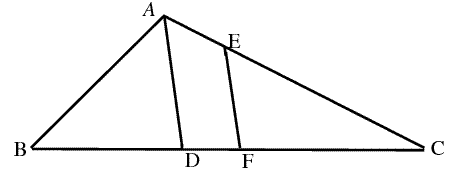1. Let $$m,n$$ be positive integers that satisfy $$(m+1)^3-m^3=n^2$$. Prove that there exist $$k\in\mathbb{N}$$ such that $$k^2+(k+1)^2=n$$.

2. In the following picture, AD bisect $$\angle_{BAC}$$, |BF|=|FC|, EF parallel to AD. If |AB|=7, |AC|=11, prove that |EC| is an odd integer.More problems are available in the actual course and you can request for solutions there. You can also post your own problems.